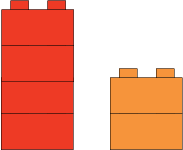# Math Example--Measurement--Comparing Heights--Example 7

This is part of a collection of math examples involving height comparisons. These downloadable math examples can easily be incorporated into a lesson plan.

### To see the complete collection of math examples on the topic of comparing measurements, click on this link.

The following section includes background information on the topic of measurement and inequalities. This includes a video (with transcript).

Watch this video to learn about comparing heights. (The transcript is also included.)

## Comparing Heights

In this video see examples of height comparison and inequalities.The video was uploaded on 10/13/2022.

You can view the video here.

The video lasts for 2 minutes and 9 seconds.

Video Transcript

You can compare the measurements of two objects without having to take any measurements.

In cases like this, use inequality symbols.

Here are two pens, A and B.

By placing the pens side by side, like this, you can tell that A is longer than B without knowing the exact lengths of A and B.

If we let the letters represent the lengths, then we can write these inequalities:

A > B or B < A.

Here are more examples.

Here is a scale for comparing weights.

Item B is heavier than item A.

If we let the letters represent the weights, then you can write this inequality.

B > A or A < B.

Now let's use this idea to compare different heights.

Height is how tall something is.

Like length, heights can be compared by putting two or more items side by side.

Here are three plants, each labeled with a letter.

If we let the letters represent the height of each plant, then we can arrange the heights from shortest to tallest using this inequality.

B < C < A.

In order from tallest to shortest, you can use this inequality.

A > C > B.

Now let's look at an example of height measurements using numbers.

A person's height can be measured in feet and inches.

Here are three students and their heights are shown in inches.

We compare the heights by comparing the numbers.

Use place value to sort the numbers from least to greatest.

48 < 52 < 54.

Using a number line helps with comparing measurements.

As you can see, in going from left to right the values increase.

When you see numbers graphed on a number line, their relative position tells you which values are greater than others.

## Comparisons Involve Numbers

When comparing two quantities, the comparison usually comes down to comparing two numbers. Inequality symbols are used to compare numbers. Here are some examples.

### 0.5 < 1.7

Comparisons sometimes involve quantities that are equal:

## Measurements Involve Numbers

Whether you’re measuring time, distance, temperature, or whatever, the measurements involve numbers. Here are some examples.

### A temperature of 75 degrees

A measurement always includes a unit of measurement. Units of measurement include degrees, inches, feet, and any other way of getting a numerical measurement.

## Comparing Measurements

When comparing measurements, make sure the quantities are in the same unit of measurement. Take a look at this example:To compare these two measurements, use the same units of measurements. Express both measurements in inches and then compare.## Using “Units” for Measurements

You can also measure quantities using the objects themselves as a unit of measure. For example, you can compare the heights of stacks of Legos by simply counting the Legos and comparing.In this example, each Lego is a single unit of measurement.

## Using Standard Units of Measurements

We don't normally use Legos to measure heights. For one thing, Legos can only measure in whole units, not half or quarter units. To get precise measurements we need measurement tools that have agreed-upon measurements and can measure objects in fractional amounts. This is why rulers that measure in inches or millimeters provide standard and accurate ways of measuring.

When comparing measurements, keep the following in mind:

• Make sure the measurements use the same unit of measurements.
• If necessary convert one of the measurement units so that the same units of measurements are being compared.
• The comparison only involves the numbers.
• Look for equivalent measurements. Some measurements are equal, even if they may not look that way initially.

The downloadable image is part of a collection of math examples that focus on measurement.